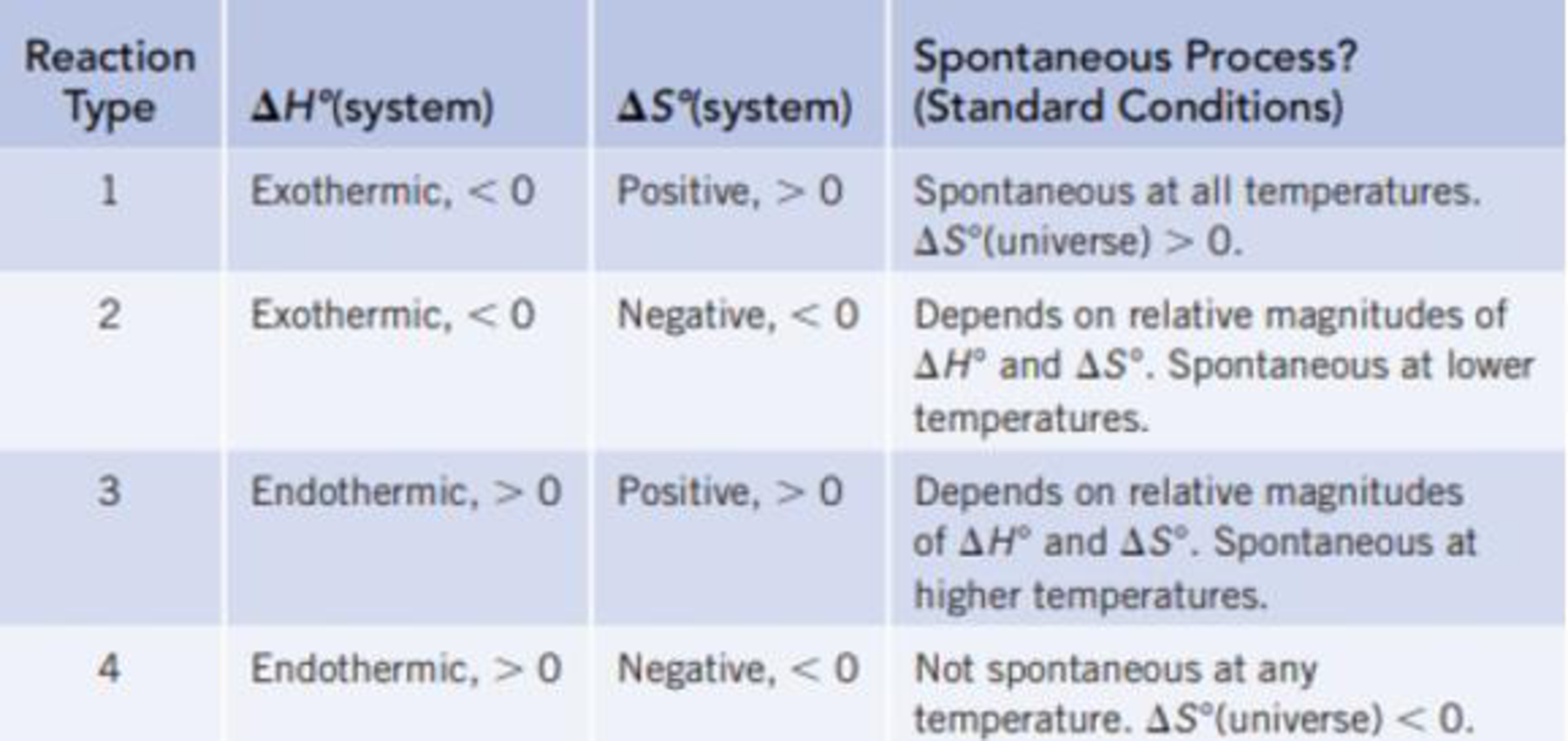Chapter 18, Problem 29PS

Chapter
Section
Textbook Problem

Classify each of the reactions according to one of the four reaction types summarized in Table 18.1. (a) Fe2O3(s) + 2 Al(s) → 2 Fe(s) + Al2O3(s) ΔrH° = −851.5 kj/mol-rxn ΔrS° = −375.2 J/K · mol-rxn (b) N2(g) + 2 O2(g) → 2 NO2(g) ΔrH° = 66.2 kJ/mol-rxn ΔrS° = −121.6 J/K · mol-rxn TABLE 18.1 Predicting Whether a Reaction Will Be Spontaneous Under Standard Conditions(a)

Interpretation Introduction

Interpretation: The reaction Fe2O3(s)+2Al(s)2Fe(s)+Al2O3(s) should be classified based on reaction types discussed in Table 18.1 on the basis of given ΔS and ΔH values.

Concept introduction: The universe consists of two parts, systems and surroundings. The entropy change for the universe is the sum of entropy change for the system and for surroundings.

ΔS(universe)= ΔS(system)+ΔS(surroundings)

The ΔS(universe) should be greater than zero for a spontaneous process.

The  ΔS(system) can be calculated by the following expression,

ΔS(system)=ΔrS°=nS°(products)nS°(reactants)

The ΔS(surroundings) can be calculated by the following expression,

ΔS(surroundings)=ΔrHT

Here, ΔrH is the enthalpy change for the reaction.

Thus, a reaction can be categorized as spontaneous or non-spontaneous on the basis of ΔS and ΔH values.

Explanation

Referring Table 18.1 the given reaction classified as follows,

The given reaction is,

Fe2O3(s) + 2Al(s)2Fe(s) + Al2O3(s)

The value of ΔrH is 851.5 kJ/mol- rxn

(b)

Interpretation Introduction

Interpretation: The reaction N2(g) + 2O2(g)2NO2(g) should be classified based on reaction types discussed in Table 18.1 on the basis of given ΔS and ΔH values.

Concept introduction: The universe consists of two parts, systems and surroundings. The entropy change for the universe is the sum of entropy change for the system and for surroundings.

ΔS(universe)= ΔS(system)+ΔS(surroundings)

The ΔS(universe) should be greater than zero for a spontaneous process.

The  ΔS(system) can be calculated by the following expression,

ΔS(system)=ΔrS°=nS°(products)nS°(reactants)

The ΔS(surroundings) can be calculated by the following expression,

ΔS(surroundings)=ΔrHT

Here, ΔrH is the enthalpy change for the reaction.

Thus, a reaction can be categorized as spontaneous or non-spontaneous on the basis of ΔS and ΔH values.

Still sussing out bartleby?

Check out a sample textbook solution.

See a sample solution

The Solution to Your Study Problems

Bartleby provides explanations to thousands of textbook problems written by our experts, many with advanced degrees!

Get Started

Find more solutions based on key concepts